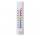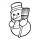# Temperature + integers - math problemsIn about 12 hours in North Dakota the temperature rose from -33 degrees farenheit to 50 degrees farenheit. By how much did the temperature change?
2. The temperatureThe temperature at 1:00 was 10 F. Between 1:00 and 2:00, the temperature dropped 15F. Between 2:00 and 3:00, the temperature rose 3F. What is the temperature at 3:00?
3. Degrees 2The temperature was 3°F and falls four degrees Fahrenheit. What is actually temperature?
4. Thermometerthermometer showed -12 degrees Celsius at the morning then the temperature rises by 4 degrees and later again increased by 2 degrees at the evening has fallen by 5 degrees and then fall 3 degrees. What end temperature does the thermometer show?
5. FreezerTemperature is -8 degrees Celsius in the freezer. Outside temperature is 2 degrees Celsius. One minute took to change temperature by one degree Celsius if we open door. How many minutes the temperature in the freezer will rise?
6. ThermometerThe thermometer showed -3°C at morning. Then temperature was increased by 1°C again increased by 1°C and then decreased by 1°C and then decreased by 4°C. Which terminal temperature thermometer shows?

We apologize, but in this category are not a lot of examples.
Do you have an interesting mathematical word problem that you can't solve it? Enter it, and we can try to solve it.

We will send a solution to your e-mail address. Solved examples are also published here. Please enter the e-mail correctly and check whether you don't have a full mailbox.

Please do not submit problems from current active competitions such as Mathematical Olympiad, correspondence seminars etc...